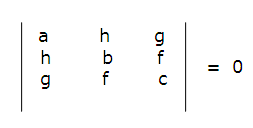# Geometry

#### Coordinate Geometry - 2

Coordinate Geometry - 2

Circles And Pair of Straight Lines :

A circle is the locus of a point which is at a fixed distance from a fixed point. This fixed point is known as centre and the fixed distance as radius. Let us say that the centre of a circle is (h,k) and its radius is ‘r’ units and let (x,y) be any point on the circumference of the circle .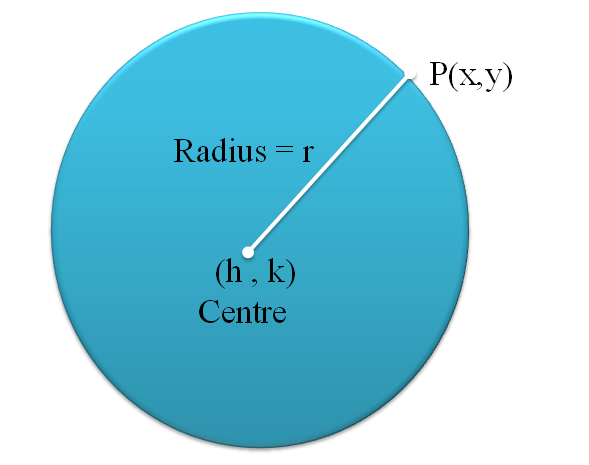Squaring both sides, we get (x-h)2 + (y-k)2 = r2  and this is the equation of a circle with centre (h,k) and radius r units.

If the centre lies at the origin, then h =0 and k =0, so equation of circle becomes:

x2 + y2 = r2

General form of equation of a Circle:

The second degree equation representing circle is given by :

x2 + y2 + 2gx + 2fy +c =0, where

Coordinates of centre are (-g,-f)Equation of a circle with opposite diametric points: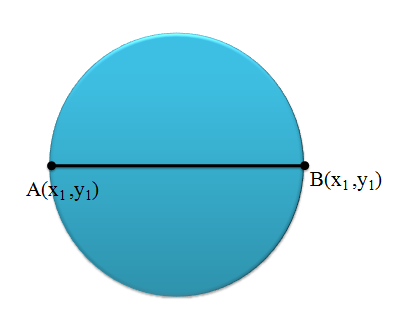If (

If (x1,y1) and (x2,y2) are the diametric points of a circle, then the equation of the circle is given by :

(x-x1)(x-x2) + (y-y1)(y-y2) = 0

Example 1: Find the area of a circle lying in the first quadrant touching both the axes and passing through the point (4,2).

Solution : The circle lies in the first quadrant and touches both the axes.

So, centre becomes C(r,r) and radius is ‘r’ units

Equation of the circle becomes: (x-r)2 + (y-r)2 = r2

Or  x2 + r2 -2xr + y2 + r2 -2yr = r2

x2 +y2 -2r(x+y) + r2 = 0

The circle passes through the point (4,2).

So,    42 + 22 -2r(4+2) + r2 = 0

r2 -12r + 20 = 0

Thus, r = 10 or 2 units

So, either the area of the circle is 4p or 100p.So, either the area of the circle is 4π or 100π.

Intercepts Made by a Circle :

Let us say that a circle C1 cuts the X axis at two points A and B as shown in the figure. Definitely as the points lie on the X axis, so their Y coordinates are zero and let x1 and x2 be their x coordinates respectively.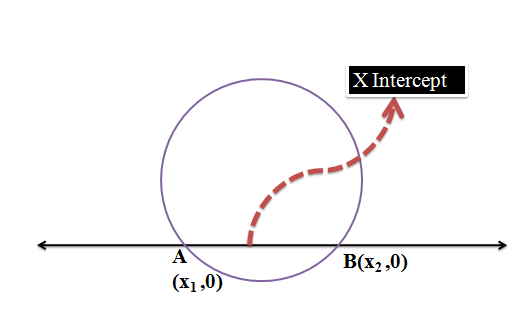So, X intercept is basically the portion cut by the circle on the X axis. As y =0, putting it in the equation of circle, we get a quadratic in x only whose roots are x1 and x2 . The difference between the two roots will give us the length of the X intercept.

Let’s say the equation of circle is x2 +y2 + 2gx + 2fy + c = 0. If we put y=0 in this equation, we get a quadratic : x2 +2gx + c = 0 whose roots are x1 and x2

(x2-x1)2 = (x1+x2)2 – 4x1x2

= (-2g)2 -4c  = 4(g2-c)Example 2: Find the sum of the intercepts formed by the circle x2 +y2 +10x +11y +24 =0 with both the axes.

Solution: X intercept is made by the points where the circle intersects the X axis and similarly Y intercept is made by the points where the circle intersects the Y axis.

Calculation of X intercept

For the points where the circle intersects the X axis, ordinate is zero. So,

x2 + 10x +24 = 0

Roots are -6,-4

X intercept = -4-(-6) = 2 units

Calculation of Y intercept

For the points where the circle intersects the Y axis, abscissa is zero. So,

y2 + 11x +24 = 0

Roots are -8,-3

Y intercept = -3-(-8) = 5 units

Sum of X intercept and Y intercept = 2+5 = 7 units.

Example 3: Check whether the circle x2 + y2 + 8x +6y +18=0  forms X intercept and Y intercepts. If yes then calculate their lengths.

Solution: Equation of the circle: x2 + y2 + 8x +6y +18=0

X intercept:

Put y =0, we get x2 + 8x + 18

As the discriminant is negative, we get imaginary roots and so this equation does not have real roots. So, the circle doesn’t intersect the X axis. And so, we do not get any X intercept.

Y intercept:

Put x = 0, we get x2 + 6y +18

As the discriminant is negative, we get imaginary roots and so this equation does not have real roots. So, the circle doesn’t intersect the Y axis. And so, we do not get any Y intercept.

Example 4: For a triangle circumscribed in a circle, the co-ordinates of two vertices of the triangle are A(3,7) and  B(9,7).Find the co-ordinates of the third point on the x axis and the circle, given that A and B lie on the diameter of the circle.

Solution:

Since the point lie on the x-axis hence the y co-ordinate of the point is zero.

Also, angle BCA=900

slope of BC * slope of AC = -1x2 +2x-15 = 0

(x+5)(x-3) = 0

Or x = -5 and x = 3

Thus, the coordinates of the third point are (-5,0) or (3,0)

Condition for a line to be a Tangent:

If a line is tangent to a circle, then the distance to the centre from the line must be equal to the radius of the circle.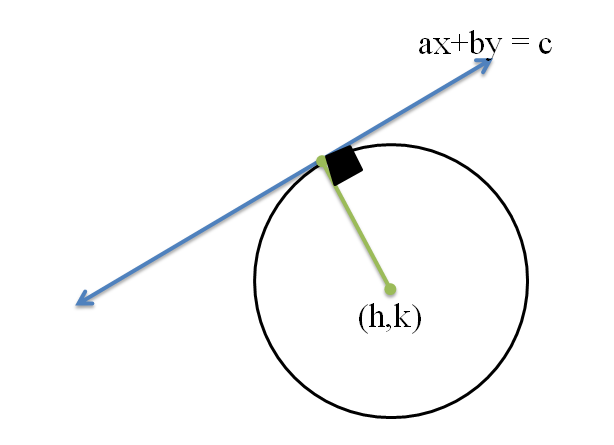Example 5: If the line 5x -12y + k =0 is a tangent to a circle x2 +y2 -2x + 4y -11 = 0, then the possible values of k are –

Solution: The equation of circle is: x2 +y2 -2x + 4y -11 = 0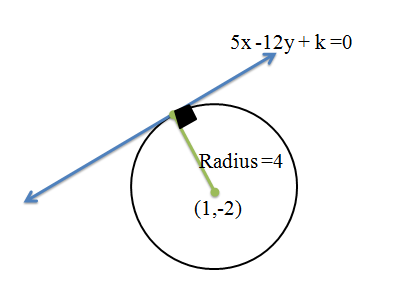Distance of the centre from the tangent must be equal to the radius of the circleOr  (29-k)/13 = ±4

29 –k = 52     or   29 –k = -52

k = -23  or k = 81

Example 6:  From a point P(-4,-5) a tangent is drawn to a circle x2 +y2 -4x -6y -23 =0. What is the length of the tangent?

Solution: Equation of circle: x2 +y2 -4x -6y -23 =0

Centre (2,3)  and radius = 6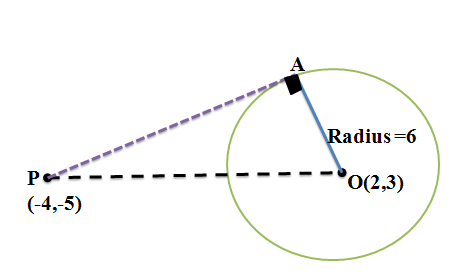Consider ?OAP, using Pythagoras theorem we get PA = 8 units

Length of tangent = 8 units.Family of Circles:

Family of circles is a set of circles which satisfy a common property.

Now, from two points, one can draw infinite number of circles passing through them.

Let us say, we have two circles whose equations are S1 and S2, then the equation of all the circles passing through the points of intersection of S1 and S2 is given by S1 + λS2

Example 7: Find the equation of a circle passing through the points of intersection of the two circles x2 +y2 -6x -2y -15 = 0 and x2 + y2 -18x -18y +126 = 0 and also through the point (0,1).

Solution:  S1: x2 +y2 -6x -2y -15 = 0 and S2 : x2 + y2 -18x -18y +126 = 0

S3 is a circle passing through the intersection of S1 and S2, so

S3= S1 + λS2 = x2 +y2 -6x -2y -15 +λ(x2 + y2 -18x -18y +126) = 0

It also passes through (0,1)

So, put this point in the equation of S3 to get the value of λ

1-2-15+ λ-18 λ+126 λ = 0  or λ = 109/16

Put this value of λ to get the final equation of S3.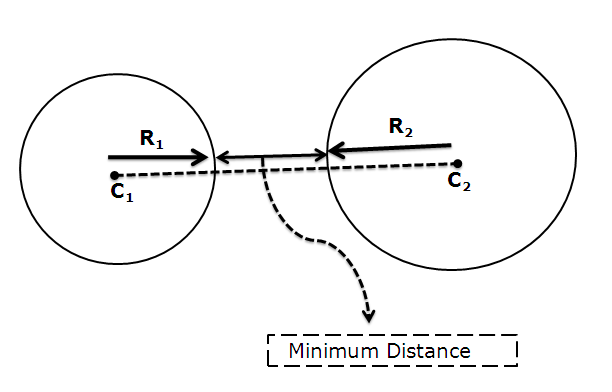Equation of Common Chord between two circles:

If S1 and S2 are two circles which intersect each other at A and B respectively, then the equation of the common chord to both these circles is given by S1 –S2 =0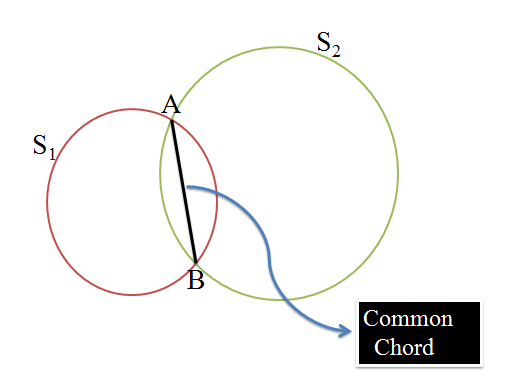Pair of Straight Lines:

The second degree equation ax2 + 2hxy + by2 + 2gx + 2fy + c = 0 represents  a pair of straight lines if: